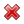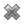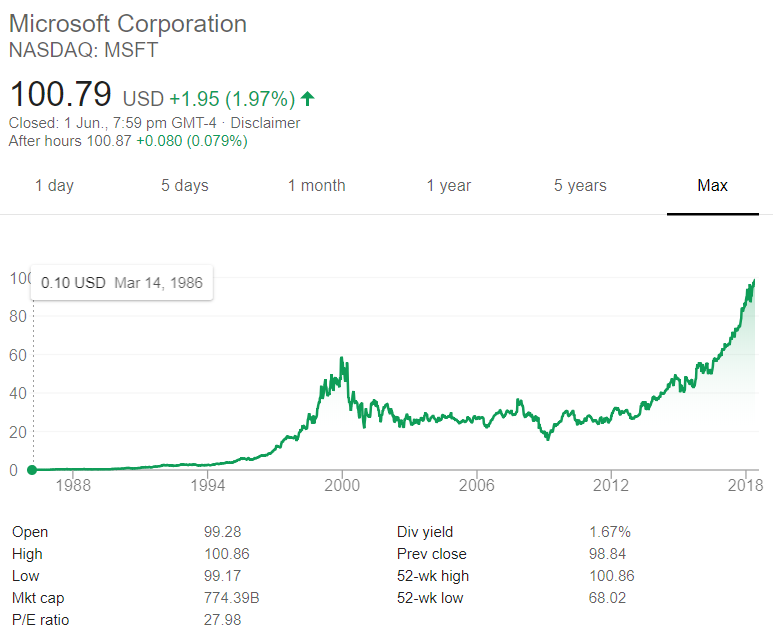# Fight Finance

#### CoursesTagsRandomAllRecentScoresScoreskeithphw $6,011.61 Jade$1,815.80 Chu $789.98 royal ne...$750.00 Leehy $713.33 Visitor$650.00 ZOE HY $640.00 JennyLI$625.61 Visitor $590.00 Visitor$555.33 Visitor $550.00 Visitor$550.00 Visitor $540.00 Visitor$500.00 Yizhou $489.18 Visitor$480.00 Visitor $470.00 Visitor$464.70 Jasper.sun $460.00 Visitor$460.00

A share just paid its semi-annual dividend of $10. The dividend is expected to grow at 2% every 6 months forever. This 2% growth rate is an effective 6 month rate. Therefore the next dividend will be$10.20 in six months. The required return of the stock is 10% pa, given as an effective annual rate.

What is the price of the share now?

You're advising your superstar client 40-cent who is weighing up buying a private jet or a luxury yacht. 40-cent is just as happy with either, but he wants to go with the more cost-effective option. These are the cash flows of the two options:

• The private jet can be bought for $6m now, which will cost$12,000 per month in fuel, piloting and airport costs, payable at the end of each month. The jet will last for 12 years.
• Or the luxury yacht can be bought for $4m now, which will cost$20,000 per month in fuel, crew and berthing costs, payable at the end of each month. The yacht will last for 20 years.

What's unusual about 40-cent is that he is so famous that he will actually be able to sell his jet or yacht for the same price as it was bought since the next generation of superstar musicians will buy it from him as a status symbol.

Bank interest rates are 10% pa, given as an effective annual rate. You can assume that 40-cent will live for another 60 years and that when the jet or yacht's life is at an end, he will buy a new one with the same details as above.

Would you advise 40-cent to buy the or the ?

Note that the effective monthly rate is $r_\text{eff monthly}=(1+0.1)^{1/12}-1=0.00797414$

If the AUD appreciates against the USD, the American terms quote of the AUD will or ?

A European call option will mature in $T$ years with a strike price of $K$ dollars. The underlying asset has a price of $S$ dollars.

What is an expression for the payoff at maturity $(f_T)$ in dollars from having written (being short) the call option?

This annuity formula $\dfrac{C_1}{r}\left(1-\dfrac{1}{(1+r)^3} \right)$ is equivalent to which of the following formulas? Note the 3.

In the below formulas, $C_t$ is a cash flow at time t. All of the cash flows are equal, but paid at different times.

An investor wants to make a portfolio of two stocks A and B with a target expected portfolio return of 12% pa.

• Stock A has an expected return of 10% pa and a standard deviation of 20% pa.
• Stock B has an expected return of 15% pa and a standard deviation of 30% pa.

The correlation coefficient between stock A and B's expected returns is 70%.

What will be the annual standard deviation of the portfolio with this 12% pa target return?

The price of gold is currently $700 per ounce. The forward price for delivery in 1 year is$800. An arbitrageur can borrow money at 10% per annum given as an effective discrete annual rate. Assume that gold is fairly priced and the cost of storing gold is zero.

What is the best way to conduct an arbitrage in this situation? The best arbitrage strategy requires zero capital, has zero risk and makes money straight away. An arbitrageur should sell 1 forward on gold and:

A 2-year futures contract on a stock paying a continuous dividend yield of 3% pa was bought when the underlying stock price was $10 and the risk free rate was 10% per annum with continuous compounding. Assume that investors are risk-neutral, so the stock's total required return is the risk free rate. Find the forward price $(F_2)$ and value of the contract $(V_0)$ initially. Also find the value of the contract in 6 months $(V_{0.5})$ if the stock price rose to$12.

If a firm makes a profit and pays no dividends, which of the following accounts will increase?

The below graph shows the computer software company Microsoft's stock price (MSFT) at the market close on the NASDAQ on Friday 1 June 2018.Based on the screenshot above, which of the following statements about MSFT is NOT correct? MSFT's: